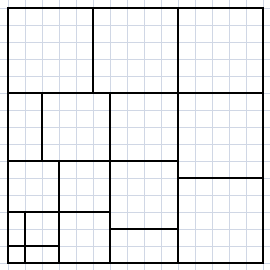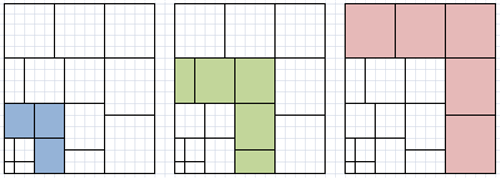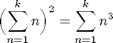## Loren on the Art of MATLABTurn ideas into MATLAB

Note

Loren on the Art of MATLAB has been archived and will not be updated.

# Nicomachus’s Theorem

Ned Gulley is stepping in as guest blogger this week. You may remember an earlier guest entry from him on calendars and leap years. Ned has been working at MathWorks almost as long as I have, and as we were chatting the other day, he reminded me of an episode from long ago.

### Nicomachus's Theorem

One day, not long after I started working at MathWorks, Loren presented me with a puzzle. She gave me a piece of graph paper with some lines drawn on it so it looked something like this.Then she asked: What mathematical statement is this making?

It's an appealing pattern and a curious question, so I took it up with great interest. After a while, I worked it out. Do you see it?

The first thing I noticed is that the block length along the bottom and left edges increases like so: 1, 2, 3, 4, and so on. Let's call this the edge sequence. It looks like we can interpret the blocks in terms of the extra area added with each number in the edge sequence. When the edge sequence reaches 3, then three blocks of size 3x3 are added to the area (blue). Similarly with 5, there are five blocks of size 5x5 (red). Four follows the same pattern, provided we stitch together the two rectangles along the edges (green).More generally, for every number n that we add to the edge sequence, we add an area equal to n squares of size nxn. ThusI thought that picture was a beautiful way to say a beautiful thing that I hadn't known before, and I always remembered it. This result is sometimes known as Nicomachus's theorem. Stated compactly, the square of the nth triangular number is equal to the sum of the first n cubes.

Since this is a MATLAB blog, I should include some code. Let's represent the left-hand side using the symsum like so (after creating a few symbolic objects).

syms n k
nsqr = symsum(n,n,1,k)^2
nsqr =
(k^2*(k + 1)^2)/4


The right-hand side, which is the sum of the first k cubes, looks like this.

ncube = symsum(n^3,n,1,k)
ncube =
(k^2*(k + 1)^2)/4


Are they the same?

isequal(nsqr, ncube)
ans =
1


Yes! So the premise appears to be true.

I was reminded of Loren's visual proof by a post that my fellow MathWorker Matt McDonnell tweeted about a few weeks ago: Six Visual Proofs.

A little more rooting around turned up this book, which looks like it would be fun if you, like me, are tickled by wholly visual proofs. Proofs without Words: Exercises in Visual Thinking by Roger B. Nelsen

Are you aware of any other nice visual proofs out there? Tell us here.

Published with MATLAB® 7.9

### 댓글

댓글을 남기려면 링크 를 클릭하여 MathWorks 계정에 로그인하거나 계정을 새로 만드십시오.